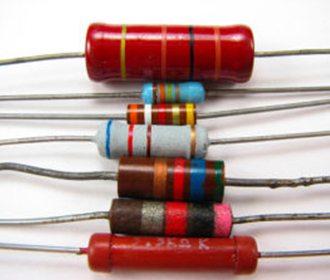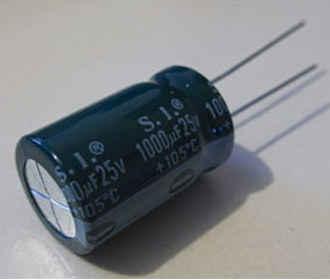News Group
Dingfeng Capacitor--Difference between Capacitor and Resistor
Issue Time:2019-05-08
Difference between Capacitor and Resistor## What is Resistor?

Resistors are fundamental components in electrical and electronic circuits used to control voltage and current values in circuits. They are basically energy-consuming elements that limit the flow of current to have the right amount of current and voltage that you want precisely in your circuits. A resistor is used where more current is required to be impeded so that the desired amount of current is achieved without any resistance. They are passive two-terminal components that implement resistance to reduce the flow of current to a safe value. They convert electrical energy into heat, which is then dissipated into the air. Resistors are generally classified into two types: fixed and variable. Resistance is measured in “Ohm”.## What is Capacitor?

Capacitors are created out of two metal plates with an insulator between them. It’s one of the fundamental passive components used in electrical circuits and what makes them so special is their ability to store energy. They store potential energy in an electric field and give it back to the circuit when required. Each capacitor is built to have a specific amount of capacitance, which specifies how much electric charge it can store. Capacitance is measured in “Farads” and is abbreviated as “F”. The purpose of a capacitor is to resist sudden changes in voltage by supplying energy to a circuit.
Difference between Capacitor and Resistor
Basics of Capacitor and Resistor:
Capacitor and resistor are the two most common basic components used in electronic circuits with each can be described in terms of the relationship between the current flow and the voltage across the component. Both are energy storage components, but they differ in the way they store energy. A resistor is an electronic component used to resist the flow of current in a circuit. It’s more like a friction which restricts energy. A capacitor, on the other hand, is an electronic component used to store electrical charge. It generally opposes changes in current in electrical and electronic circuits.
Working of Capacitor and Resistor:
A resistor is a passive two-terminal electrical component that implements controlled amounts of resistance into electrical circuits meaning it restricts the amount of current flowing through a device. It doesn’t do anything actively to any electronic circuit; in fact, it’s simply hooked into a circuit to have the currents and voltages that you want precisely in your circuit. A capacitor, on the other hand, stores potential energy in an electric field and gives it back to the circuit when required. Simply put, it charges and discharges the electric charge stored in the circuit.

Function of Capacitor and Resistor:
A resistor is a little resistance package which controls the flow of current to other components in an electrical circuit. It’s not only used to amplify signals but to limit the flow of current, adjust signal levels, terminate transmission lines, etc. It limits the current flow to a safe value. A capacitor consists of two or more parallel conductor plates with an insulator between them. The function of a capacitor is to keep the positive and negative charges separated from each other. The effect of a capacitor is known as capacitance.